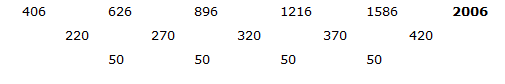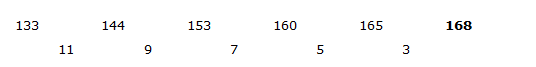# SBI Clerk Prelims 2021 Quantitative Aptitude Questions (Day-65)

Dear Aspirants, Our IBPS Guide team is providing new series of Quantitative Aptitude Questions for SBI Clerk Prelims 2020 so the aspirants can practice it on a daily basis. These questions are framed by our skilled experts after understanding your needs thoroughly. Aspirants can practice these new series questions daily to familiarize with the exact exam pattern and make your preparation effective.

Start Quiz

Time and work

1) A and B together can complete the work in 30 days. If B alone complete 20% of the work in 16 days. In how many days A alone complete 75% of the work?

A.40 days

B.36 days

C.32 days

D.28 days

E.None of these

Partnership

2) A and B started the business with the initial investments Rs.5000 and Rs.10000 respectively. After 4 months A adds Rs.1000 for his initial investment and B withdrew Rs.1500 for his initial investment. If the difference between the profit share by A and B is Rs.6480, then what is the total profit of the business?

A.Rs.8000

B.Rs.9000

C.Rs.12600

D.Rs.15800

E.Cannot be determined

SI AND CI

3) Shon invests Rs.8000 in scheme A which offer simple interest at 15% per annum for x years. After 8 years Shon received the total amount of Rs.17600. Find the value of x?

A.6 years

B.7 years

C.8 years

D.10 years

E.None of these

Boat and stream

4) Ratio of the speed of boat in still water to stream is 4: 1. If the boat rowing the speed of 20 kmph in still water, then what is the ratio of the speed of boat rowing against current and along with current?

A.2: 3

B.3: 2

C.3: 5

D.5: 3

E.None of these

Time, speed and distance

5) A car covered a distance of 840 km in 14 hours. Ratio of the speed of bike to speed of the car is 4: 3 and the ratio of the speed of bus to bike is 1: 2. In how much distance will the bus cover in 8 hours?

A.240 km

B.320 km

C.400 km

D.160 km

E.560 km

Missing number series

Direction (6-10): What should come in place of question mark in the following series?

6) 32, 80, 280, 1260, 6930,?

A.45111

B.45165

C.45045

D.45220

E.44904

7) 406, 626, 896, 1216, 1586, ?

A.2006

B.2146

C.2046

D.1956

E.1996

8) 22, 33, 46, 61, 78, ?

A.68

B.97

C.24

D.51

E.109

9) 262144, 32768, 4096, 512, 64, ?

A.3

B.13

C.32

D.8

E. 22

10) 133, 144, 153, 160, 165, ?

A.168

B.186

C.295

D.248

E.196

A + B = 1/30

B = 5/1 * 16 = 80 days

A = 1/30 – 1/80

A = (8 – 3)/240 = 5/240

A = 1/48

A complete the 75% of the work in = 48 * ¾ = 36 days

Total time period is not given.

Hence, the question cannot be answered.

SI = (P * N * R)/100

SI = 17600 – 8000 = Rs.9600

9600 = (8000 * 15 * x)/100

x = 8 years

Speed of stream = ¼ * 20 = 5 kmph

Speed of the boat along with stream = 20 + 5 = 25 kmph

Speed of the boat against the current = 20 – 5 = 15 kmph

Required ratio = 15: 25

= 3: 5

Distance = Speed * Time

Speed of car = 840/14 = 60 kmph

Speed of the bike = 4/3 * 60 = 80 kmph

Speed of the bus = ½ * 80 = 40 kmph

Distance covered by in 8 hours = 40 * 8 = 320 km

The pattern is as follows:

32 * 2.5 = 80

80 * 3.5 = 280

280 * 4.5 = 1260

1260 * 5.5 = 6930

6930 *6.5 = 45045262144/8 = 32768

32768/8 = 4096

4096/8 = 512

512/8 = 64

64/8 = 8